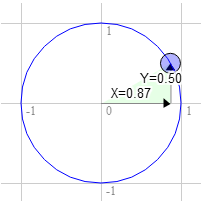# WebGLFundamentals.org

Fix, Fork, Contribute

# WebGL 二维旋转

``````<script id="vertex-shader-2d" type="x-shader/x-vertex">
attribute vec2 a_position;

uniform vec2 u_resolution;
uniform vec2 u_translation;
+uniform vec2 u_rotation;

void main() {
+  // 旋转位置
+  vec2 rotatedPosition = vec2(
+     a_position.x * u_rotation.y + a_position.y * u_rotation.x,
+     a_position.y * u_rotation.y - a_position.x * u_rotation.x);

// 加上平移
*  vec2 position = rotatedPosition + u_translation;
``````

``````  ...

+  var rotationLocation = gl.getUniformLocation(program, "u_rotation");

...

+  var rotation = [0, 1];

...

// 绘制场景
function drawScene() {

...

// 设置平移
gl.uniform2fv(translationLocation, translation);

+    // 设置旋转
+    gl.uniform2fv(rotationLocation, rotation);

// 绘制几何体
var primitiveType = gl.TRIANGLES;
var offset = 0;
var count = 18;  // 6 个三角形组成 'F', 每个三角形 3 个点
gl.drawArrays(primitiveType, offset, count);
}
``````

``````rotatedX = a_position.x * u_rotation.y + a_position.y * u_rotation.x;
rotatedY = a_position.y * u_rotation.y - a_position.x * u_rotation.x;
`````````   3.0 * 0.87 + 9.0 * 0.50 = 7.1
9.0 * 0.87 - 3.0 * 0.50 = 6.3
``````   3.0 * 0.50 + 9.0 * 0.87 = 9.3
9.0 * 0.50 - 3.0 * 0.87 = 1.9
```

``````function printSineAndCosineForAnAngle(angleInDegrees) {
var angleInRadians = angleInDegrees * Math.PI / 180;
console.log("s = " + s + " c = " + c);
}
``````

``````  ...
var angleInRadians = angleInDegrees * Math.PI / 180;
``````

### 弧度是什么?

• 基础概念
• 图像处理
• 二维平移，旋转，缩放和矩阵运算
• 三维
• 光照
• 组织和重构
• 几何
• 纹理
• 渲染到纹理
• 阴影
• 技术
• 建议
• 优化
• 杂项
• 参考

Issue/Bug? 在GitHub上提issue.